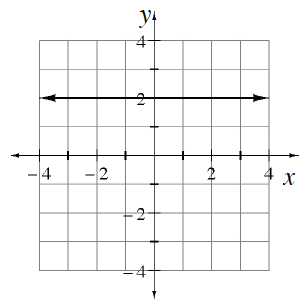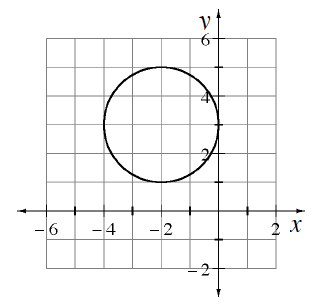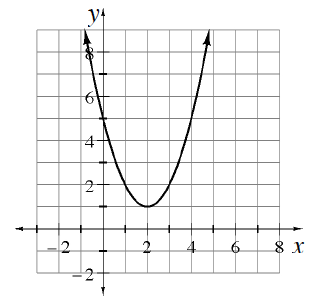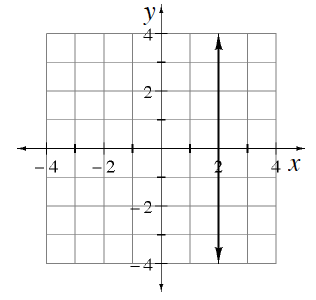### Home > INT2 > Chapter 9 > Lesson 9.3.1 > Problem9-79

9-79.

Determine whether each graph shown below represents a function.

What are the components needed for a graph to be a function?

1.Is a function.

1.Is not a function.

1.1.1. A constant function is a function where every input value gives the same output value. Are any of the relationships shown in parts (a) through (d) also constant functions? Explain why or why not